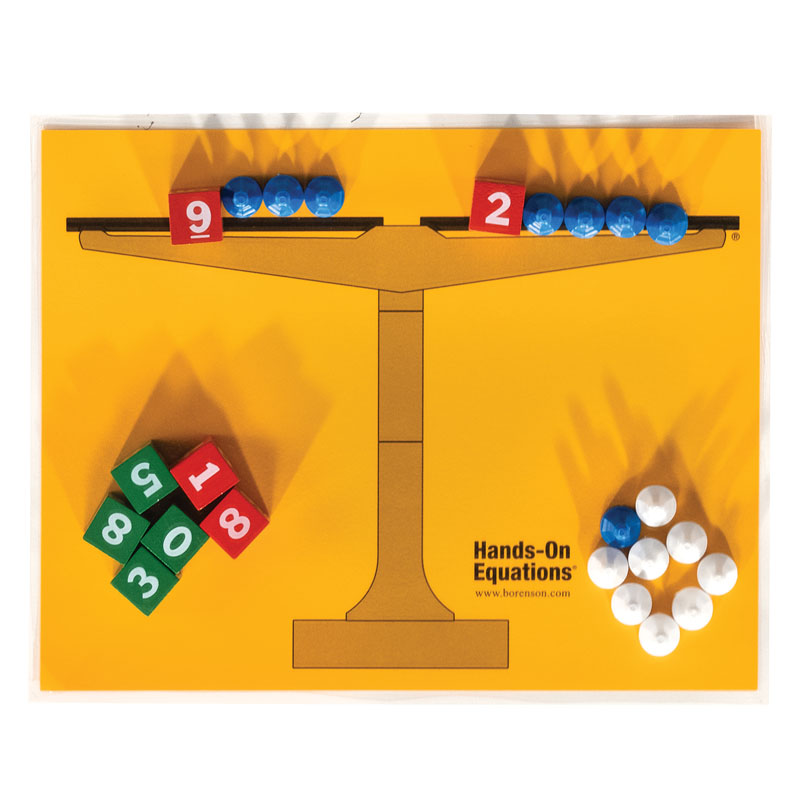# Set of Student Game Pieces and Laminated Scale

# 021796

Our Price: \$6.65
In Stock.
Qty:
Qty:

Category Description for Hands-On Equations:

Good manipulatives demonstrating algebraic concepts are hard to find. Developed by Dr. Henry Borenson, Hands-On Equations Learning System lays a concrete foundation and introduction to algebraic equations as early as third grade. Using simple manipulatives (laminated pictorial balance, pawns, and dice), this method is almost game-like, but imparts real algebraic understanding. The methodology is simple but effective, as the scale represents balance (the equal sign), pawns stand for positive and negative unknowns, and dice for the whole numbers in the equation. By using a series of legal "moves," players (students) manipulate variables and solve the equation. Use this program anytime before your child encounters textbook algebra - it will certainly simplify the understanding of textbook (pen and paper) algebra. Even older children and adults using the program have benefited from it, commenting that it finally made algebra understandable. 26 lessons progress from simple to complex, with three levels of learning. The program contains all manipulative pieces, Level I, II, and III instructional manuals, worksheets, and an answer key.

An Instructional DVD is designed to be used with these materials. It is a step-by-step visual guide to Hands-On Equations. Each of the 26 lessons is demonstrated by Dr. Borenson, Molly Richman, or Eric Borenson. You can view the lessons one at a time, and it is ideal for introduction or review of a concept. 120 minutes.

The Hands-On Equations Verbal Problems Book is for students who have completed Level 1 of the program. This book assumes that students have worked through level 1, so step-by-step solutions aren't presented. This book focuses on going from the verbal problem to the representation of the variables using the pawns and cubes of the program. There are 7 sections including a chapter on getting started, number problems, consecutive number problems, age problems, coin problems, distance problems, and story and miscellaneous problems. Problems become progressively more difficult as you move through each section. The appendix has suggestions for teaching verbal problems using the Hands-On Equations program. Also available is the Verbal Problems Introductory Workbook, which serves as a bridge between Level I and the Verbal Problems Book. The workbook contains a graduated progression of 27 lessons, assuring that students have a successful introduction to verbal problems. Helpful templates are provided with each example. Both Verbal Problems books are valuable extensions to an already invaluable program.

The Hands-On Equations Home Packet includes the Hands-On Equations Learning System, Instructional DVD, and the Verbal Problems Introductory Workbook. Together, these components will provide your student with a very comprehensive hands-on tool for algebraic comprehension.

An Additional Student Kit is available if working with more than one student. These are the same pieces included in the main program: laminated balance, pawns, and dice. This would allow students to each have their own set of manipulatives with which to work.

Now even younger children can begin to learn the basics of algebra with the Verbal Problems Introductory Workbook. This book is designed for use with children as young as 3rd grade and concepts concerning age, cost, measuring perimeter, averages, distance, and rate of travel are all covered in the scope of 27 lessons. Each lesson includes 3 problems in which the student uses the provided template to learn to solve for the missing variable. The use of this book assumes that you have completed Level I of Hands-On Equations or at least the first four lessons along with an introduction to the pictorial notation found in lesson seven. Solutions are found in the back of the book.

I wish something like this had been around during my youth. As I worked some of the problems in the books, I could literally "see" how the equations were suppose to work. ~ Donna

Primary Subject
Mathematics
3
Format
Math Manipulative
Brand Name
Borenson & Associates
Weight
0.2 (lbs.)
Dimensions
11.25" x 8.75" x 0.5"
Browse 1 question Browse 1 question and 9 answers
Why did you choose this?
Rainbow Resource Center Store
To help my student visualize algebraic equations.
on May 10, 2022
using it in sons school
on May 23, 2019
To help my student visualize algebraic equations.
on May 10, 2022
multiple students in family
on Dec 21, 2021
multiple students in family
on Dec 21, 2021
I need a second set for my other student.
on Jul 6, 2018
5.0 / 5.0
1 Review
5 Stars
4 Stars
3 Stars
2 Stars
1 Star
1
0
0
0
0
Rated 5 out of 5